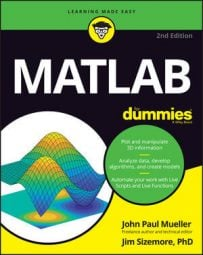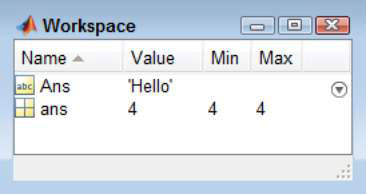##### MATLAB For DummiesComputers contain memory, much as your own brain contains memory. The computer’s memory stores information that you create using MATLAB. Looking at memory as a kind of storage locker can be helpful. You open the door, put something inside and then close the door until you need the item again. When that happens, you simply open the door and take the item out.

Whenever you tell MATLAB to store something in memory, you’re using a variable. Developers use the term variable to indicate that the content of the memory isn’t stable — it can change.

## Using ans — the default storage locker

MATLAB always needs a place to store the output of any calculation you perform. For example, when you type 2 + 2 and press Enter, the output tells you that the value is 4. However, it more specifically tells you that ans = 4. MATLAB uses ans as a storage locker when you don’t specify a specific storage locker to use.

MATLAB uses ans as a temporary storage locker. The content lasts only as long as you keep MATLAB open and you don’t perform another calculation that requires ans to hold the output. If you need the result from a calculation for additional tasks, you must store the result in another variable.

## Creating your own storage lockers

Whenever you need to use the result of a calculation in future calculations, you must create your own storage locker to hold the information; using the ans temporary variable just won’t work. Fortunately, creating your own variables is straightforward. You can create your own variables that you can use for storing any MATLAB information you want.

### Define a valid variable name

A MATLAB variable name has certain requirements, just as naming other kinds of things must meet specific requirements. Here are the rules for creating a MATLAB variable:

• Letters

• Digits

• Underscores

With this in mind, naming a variable 7Heaven doesn’t work because this particular variable name begins with a number — and variables must begin with a letter. Likewise, Doug’sStuff doesn’t work as a variable name because the apostrophe () isn’t allowed as part of a variable name. However, all the following variable names do work:

• MyVariable

• My_Variable

• My7Joys

In each case, the variable name begins with a letter and is followed by a letter, digit, or underscore. If you violate any of these rules, you see this error message:

`Error: Unexpected MATLAB expression.`

Always make variable names meaningful. Even though a variable named x is easy to type, remembering what x contains isn’t so easy. A name such as CosOutput is much easier to remember because it has meaning. At least you know that it contains the output from a cosine calculation. The more meaningful you make the name, the easier it will be for you to later determine what a calculation does.

To create your own variable, type the variable name, an equal sign, and the value you want to assign to that variable. For example, to create a variable called MyName and assign it a value of Amy, you type MyName = ‘Amy' and press Enter. (The single quotes show that Amy is a value [data], rather than another variable with the name of Amy.)

### Understand that variables are case sensitive

You need to type command and function names precisely as described in the MATLAB documentation because MATLAB is case sensitive. Variable names are also case sensitive, and this is one of the ways in which many users make mistakes when creating a script. The variable myVariable is different from MyVariable because the case is different.

### Avoid existing variable names

Avoiding the use of existing MATLAB names such as pi, i, j, sin, cos, log, and ans is essential. If you don’t know whether a particular name is in use, you can type exist(‘variable_name’) and press Enter.

Try it now with pi. Type exist(‘pi’) and press Enter. You see an output of 5, which means that the variable is in use. Now, type exist(‘MyVariable’) and press Enter. The output is 0, which means that the variable doesn’t exist.

MATLAB lets you create case-sensitive variations of existing variables. For example, type Ans = ‘Hello' and press Enter. You see that the Workspace window now displays two variables, ans and Ans. Using a variable with the same name but different capitalization as an existing MATLAB variable will cause you problems. You’re better off to simply avoid any existing term no matter how you capitalize it.